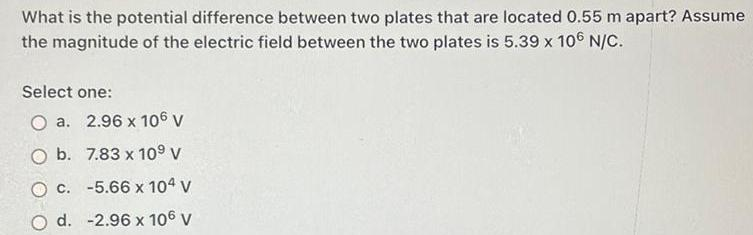Question:

# What is the potential difference between two plates that are

Last updated: 7/10/2022What is the potential difference between two plates that are located 0.55 m apart? Assume the magnitude of the electric field between the two plates is 5.39 x 10^6 N/C. Select one: a. 2.96 x 10^6 V b. 7.83 x 10^9 V c. -5.66 x 10^4 V d. -2.96 x 10^6 V